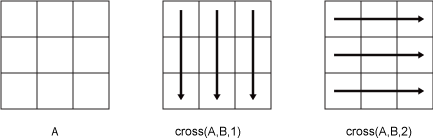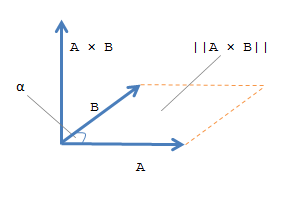# cross

## 语法

``C = cross(A,B)``
``C = cross(A,B,dim)``

## 说明

``C = cross(A,B)` 返回 `A` 和 `B` 的叉积。如果 `A` 和 `B` 为向量，则它们的长度必须为 3。 如果 `A` 和 `B` 为矩阵或多维数组，则它们必须具有相同大小。在这种情况下，`cross` 函数将 `A` 和 `B` 视为三元素向量集合。该函数计算对应向量沿大小等于 3 的第一个数组维度的叉积。`

``C = cross(A,B,dim)` 计算数组 `A` 和 `B` 沿维度 `dim` 的叉积。`A` 和 `B` 必须具有相同的大小，并且 `size(A,dim)` 和 `size(B,dim)` 必须为 3。`dim` 输入是一个正整数标量。`

## 示例

```A = [4 -2 1]; B = [1 -1 3];```

`C = cross(A,B)`
```C = 1×3 -5 -11 -2 ```

`dot(C,A)==0 & dot(C,B)==0`
```ans = logical 1 ```

`A = randi(15,3,5)`
```A = 3×5 13 14 5 15 15 14 10 9 3 8 2 2 15 15 13 ```
`B = randi(25,3,5)`
```B = 3×5 4 20 1 17 10 11 24 22 19 17 23 17 24 19 5 ```

`C = cross(A,B)`
```C = 3×5 300 122 -114 -228 -181 -291 -198 -105 -30 55 87 136 101 234 175 ```

```A = randi(10,3,3,3); B = randi(25,3,3,3);```

`C = cross(A,B,2)`
```C = C(:,:,1) = -34 12 62 15 72 -109 -49 8 9 C(:,:,2) = 198 -164 -170 45 0 -18 -55 190 -116 C(:,:,3) = -109 -45 131 1 -74 82 -6 101 -121 ```

`D = cross(A,B,3)`
```D = D(:,:,1) = -14 179 -106 -56 -4 -75 2 -37 10 D(:,:,2) = -37 -162 -37 50 -124 -78 1 63 118 D(:,:,3) = 62 -170 56 46 72 105 -2 -53 -160 ```

## 输入参数

• `cross(A,B,1)``A``B` 的列视为向量，并返回对应列的叉积。

• `cross(A,B,2)``A``B` 的行视为向量，并返回对应行的叉积。## 详细信息

### 叉积

`$\begin{array}{l}A={a}_{1}\stackrel{^}{i}+{a}_{2}\stackrel{^}{j}+{a}_{3}\stackrel{^}{k}\text{\hspace{0.17em}}\text{\hspace{0.17em}},\\ B={b}_{1}\stackrel{^}{i}+{b}_{2}\stackrel{^}{j}+{b}_{3}\stackrel{^}{k}\text{\hspace{0.17em}}\text{\hspace{0.17em}}.\end{array}$`

`$\begin{array}{l}C=A×B=|\begin{array}{cc}\begin{array}{cc}{\stackrel{^}{i}}_{}& {\stackrel{^}{j}}_{}\end{array}& {\stackrel{^}{k}}_{}\\ \begin{array}{cc}\begin{array}{c}{a}_{1}\\ {b}_{1}\end{array}& \begin{array}{c}{a}_{2}\\ {b}_{2}\end{array}\end{array}& \begin{array}{c}{a}_{3}\\ {b}_{3}\end{array}\end{array}|\\ \\ \text{\hspace{0.17em}}\text{\hspace{0.17em}}\text{\hspace{0.17em}}\text{\hspace{0.17em}}\text{\hspace{0.17em}}=\left({a}_{2}{b}_{3}-{a}_{3}{b}_{2}\right)\stackrel{^}{i}+\left({a}_{3}{b}_{1}-{a}_{1}{b}_{3}\right)\stackrel{^}{j}+\left({a}_{1}{b}_{2}-{a}_{2}{b}_{1}\right)\stackrel{^}{k}\text{\hspace{0.17em}}\text{\hspace{0.17em}}.\end{array}$`

`$‖A×B‖=‖A‖\text{\hspace{0.17em}}‖B‖\mathrm{sin}\alpha \text{\hspace{0.17em}}\text{\hspace{0.17em}}.$`